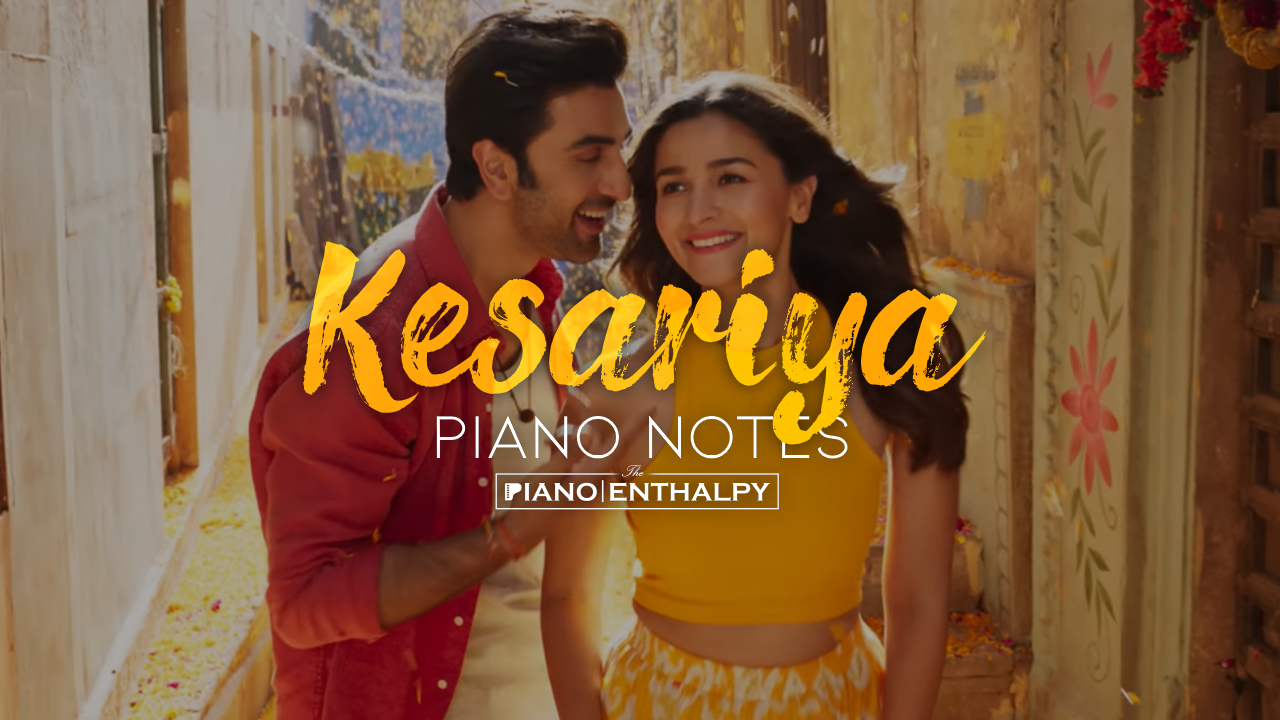# Kesariya Piano Notes (Brahmastra Part One: Shiva) | Arijit Singh | Full Song Easy Piano Notes

Song: Kesariya
Movie:
Brahmastra
Singer: Arijit Singh
Lyrics: Amitabh Bhattacharya
Music:
Pritam
Label: Sony Music IndiaKesariya Piano Notes (Brahmastra Part One: Shiva) | Arijit Singh | Full Song Easy Piano Notes

Intro:-
E+C+E+C+E+C+G C+
D+C+D+C+G+C+D+
[2 TIMES]

Mujhko
G+E+E+ F+E+D+
Itna Bataye Koyi
D+E+F+ E+D+C+BC+
Kaise Tujhse
GAG+E+E+ F+E+D+
Dil Na Lagaye Koyi
D+E+F+ E+D+C+BC+

Rabba Ne Tujhko Banane Mein
C+D+E+ G+F+E+D+ C+D+E+
Kardi Hai Husn Ki
G+F+E+D+ C+D+E+
Khaali Tijoriyan
G+F+E+D+C+D+

Kajal Ki Siyahi Se Likhi
C+D+E+ G+F+E+D+ C+D+E+
Hai Tune Jaane
G+F+E+D+C+
Kitno Ki Love Story’an
AC+D+D+E+E+ F+E+D+C+D+

Kesariya Tera
C+E+D+E+D+ C+B
Ishq Hai Piya
G+G+G+ A+G+F+ E+D+
Rang Jaaun Jo Main
D+D+BD+D+D+
Hath Lagaun
BD+E+F+E+ E+D+C+
Din Beete Saara
GA E+D+E+D+ C+B
Teri Fikr Mein
G+G+G+ A+G+F+ E+D+
Rain Saari Teri
D+D+BD+D+D+
Khair Manaun
BD+E+F+E+ E+D+C+ C+C+BD+

E+E+G+ C+D+D+D+
Rangi Chanaron Jaisi
D+D+ F+E+D+ E+E+G
Jhanke Sannnaton Mein Tu
E+E+G+ C+D+D+D+
Veena Ke Taaron Jaisi
D+D+ F+E+D+ E+E+E+
E+F+E+D+E+G+E+D+C+B D+BAG

E+E+G+ C+D+D+D+
Mann Ki Amavasein Hain
D+D+ F+E+D+ E+E+G
E+E+E+G+ C+D+D+D+
Tyoharon Jaisi
D+D+F+E+D+E+

Chanda Bhi Deewana Hai Tera
C+D+E+ G+F+E+D+ C+D+E+
Jalti Hai Tujhse
G+F+E+D+ C+D+E+
Saari Chhakoriyan
G+F+E+D+C+D+ C+D+

Kajal Ki Siyahi Se Likhi
C+D+E+ G+F+E+D+ C+D+E+
Hai Tune Jaane
G+F+E+D+C+
Kitno Ki Love Story’an
AC+D+D+E+E+F+ E+D+C+D+

Kesariya Tera
C+E+D+E+D+ C+B
Ishq Hai Piya
G+G+G+ A+G+F+ E+D+
Rang Jaaun Jo Main
D+D+BD+D+D+
Hath Lagaun
BD+E+F+E+ E+D+C+
Din Beete Saara
GA E+D+E+D+ C+B
Teri Fikr Mein
G+G+G+ A+G+F+ E+D+
Rain Saari Teri
D+D+BD+D+D+
Khair Manaun
BD+E+F+E+ E+D+C+ C+GAC+E+D+

Kesariya Tera Ishq Hai Piya
E+E+E+ E+E+ E+E+ E+ E+E+
Ishq Hai Piya
F+F+ F+ F+F+E+
Kesariya Tera Ishq Hai Piya
E+E+E+D+C+E+ E+E+E+ C+C+
Ishq Hai Piya
F+E+ D+C+BA
Kesariya
GBC+D+C+BC+

1.2.Very matching notes

3.1.No It wasnt fake just some notes didnt match !!!!

2.Yes some notes didn't match

4.Notes was good but some notes doesn't match, but thank you so much

5.Good rhythm but a little tangled notes. Best result on web.👍🏻👍🏻👏🏻👏🏻👌🏻👌🏻

6.Very beautiful and very good and comfortable to play ⭐⭐⭐⭐⭐

7.🐷🐖🐖

1.So what u dont need to be rude because u didnt like it

8.Very easy to play very wse full ✨✨✨✨✨👌👌👌👌👌

9.Very good same to same means when I played I thought that played the kesariya song in spotify